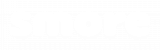# Basic Geometry Formulas

Here is the list of geometry formulas for different geometric shapes such as flat 2D shapes and 3D shapes like spheres, cones, and prisms.

Geometry deals with the different aspects of various shapes and figures. In everyday life, we apply geometry when determining the distance we have to walk from one place to another, putting together a piece of do-it-yourself furniture, or deciding whether the leftover food fits a container or not. If your student or child is new to geometry, all the shapes and formulae can be confusing. This geometry formula chart will help them see and understand instead of memorizing

## Area and Perimeter of a Circle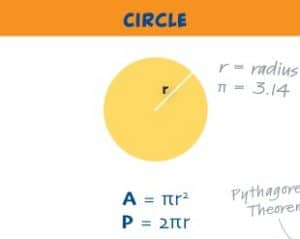Area of a Circle = πr2
Perimeter of a Circle = 2πr

## Area and Perimeter of a Right Triangle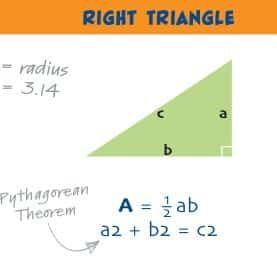Area of a Right Triangle =12 ab
Perimeter of a Right Triangle = a + b + c

## Area and Perimeter of an Equilateral Triangle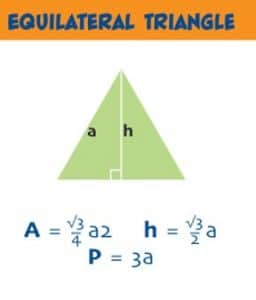Area of an Equilateral Triangle =√3/4a2
Perimeter of an Equilateral Triangle = 3a

## Area and Perimeter of a Square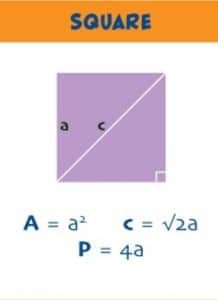Area of a Square  = a2
Perimeter of a Square = 4a

## Area and Perimeter of a Square​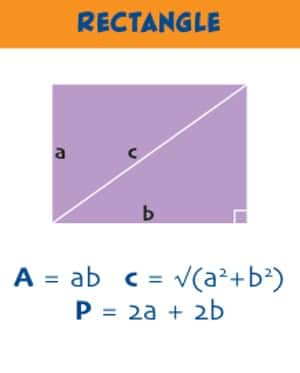Area of a Rectangle = ab
Perimeter of a Rectangle = 2a+2b

## Area and Perimeter of a Parallelogram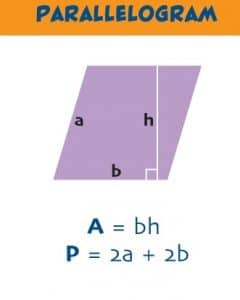Area of a Parallelogram = bh
Perimeter of a Parallelogram = 2a+2b

## Area and Parameter of a Trapezoid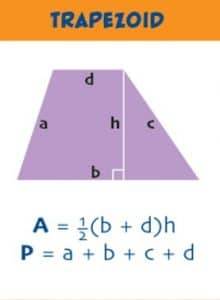Area of a Trapezoid = 1/2 (b+d)h
Perimeter of a Trapezoid = a+b+c+d

## Area and Parameter of a Pentagon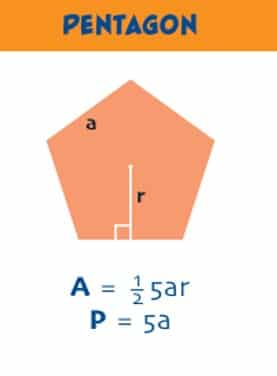Area of a Pentagon = 1/2 5ar
Perimeter of a Pentagon = 5a

## Area and Parameter of a Hexagon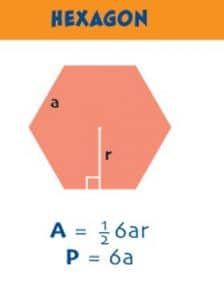Area of a Hexagon = 1/2 6ar
Perimeter of a Hexagon = 6a

## Area and Volume of a Sphere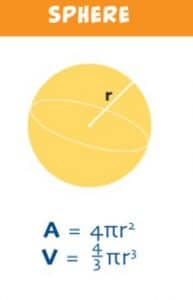Area of a Sphere = 4πr2
Volume of a Sphere = 4/3 πr3

## Area and Volume of a Cylinder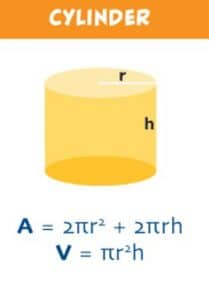Area of a Cylinder = 2πr2+ 2πrh
Volume of a Cylinder =  πr2h

## Area and Volume of a Cube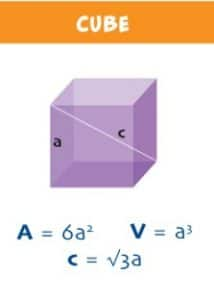Area of a Cube = 6a2
Volume of a Cube =  a3

## Area and Volume of a Cuboid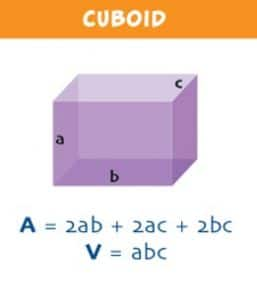Area of a Cuboid = 2ab + 2ac + 2bc
Volume of a Cuboid =  abc

## Area and Volume of a Pentagonal Prism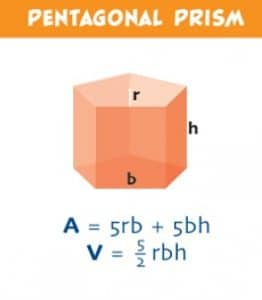Area of a Pentagonal Prism= 5rb + 5bh
Volume of a Pentagonal Prism = 5/2 rbh

## Area and Volume of a Triangular Prism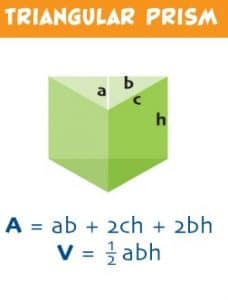Area of a Triangular Prism = ab + 2ch + 2bh
Volume of a Triangular Prism = 1/2 abh

## Area and Volume of a Cone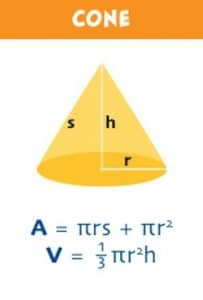Area of a Cone = πrs + πr2
Volume of a Cone = 1/3 πr2h

## Area of a Frustrum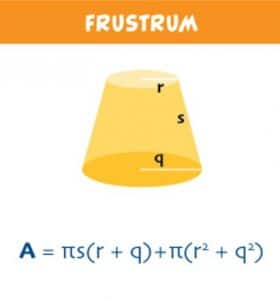Area of a Frustrum = πs(r +q) +π(r2 +q2)

## Area and Volume of a Square Pyramid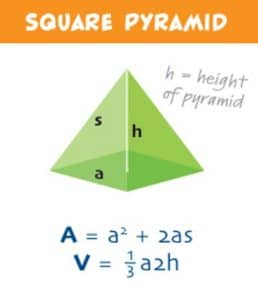Area of a Square Pyramid = a2 + 2as
Volume of a Square Pyramid =  1 /3 a2h

## Area and Volume of a Tetrahedron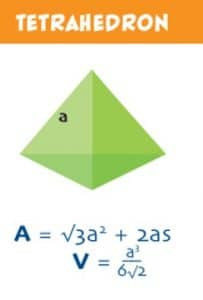Area of a Tetrahedron = √3a2 + 2as
Volume of a Tetrahedron =  a3/6√ 2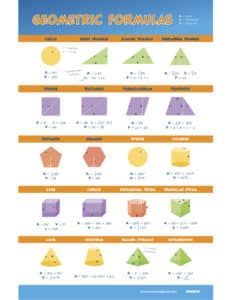All the formulas are compiled in a poster. You can download the poster here.

Join 10,000+ parents and educators
To get the FREE science digest in your inbox!

## Author

•